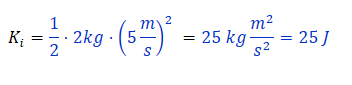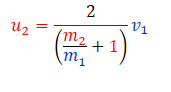top of page
Search

# Elastic Collision TutorialKey concepts:

Elastic collision

Conservation of Momentum

Conservation of Energy

From billiard balls to atoms in the air, elastic collisions are found all over the place. Let us try to figure out how they work!

Question:

"A 2 kg ball is moving with 5m/s. It collides elastically with another ball that is stationary and has a mass of 20 kg. Determine the speeds of the two balls after the collision." To get full marks, we need the following:

1. A sketch/cartoon showing the two balls before, during and after the collision.

2. The total momentum before the collision.

3. The total momentum after the collision.

4. The total kinetic energy before the collision

5. The total kinetic energy after the collision.

Let's get started with 1.

1. A sketch/cartoon showing the two balls before, during and after the collision.

To make the drawing correctly we have to remember what an "elastic collision" means. In short:

A collision is "elastic" if the objects simply bounce off of each other when they touch. The "kinetic energy" is CONSERVED!

The other case is that of "inelastic" collisions:

A collision is "inelastic" if the objects fuse together when they crash into each other. The "kinetic energy" is NOT CONSERVED!

An everyday example could be a soft snowball colliding with your face.

With this in mind, it is easy to make the drawing correctly.

Before:During:After:TIP: Always make a drawing when solving any physics problem. It makes it easier to figure out what to do in the beginning and easier to spot mistakes in your calculations!

In this problem we have defined the symbols:They represent the mass of the first ball, the mass of the second ball and the speed of the first ball respectively. Also, we have letdefine the final speeds of the two balls. These are the two quantities we want to find.

Let us get ready to solve part 2.

2. The total momentum before the collision.

The momentum before the collision can be calculated from:Think of "momentum" as meaning "the total amount of stuff that is moving". Let's look at the "before" drawing. Clearly only the mass m1 is moving at the speed v1. The other mass, m2 is stationary, soGreat! Now we can tackle 3.

3. The total momentum after the collision.

Here, we can use something called "Conservation of Momentum". This principle basically says that "The amount of stuff moving around" in the beginning is the same as "The amount of stuff moving around" in the end. Mathematically, we say that:This is a good start!

In the equation above, we only know the masses and the initial speed. We need to find both of the final speeds. To do this, we need to think about 4.

4. The total kinetic energy before the collision

Remember that kinetic energy of a single object is defined as:So the total kinetic energy before the collision must beOf course the second mass has no energy, because it is not moving initially. By plugging in the numbers we have, we find:What about the kinetic energy after? We find this in 5.

5. The total kinetic energy after the collision.

We can reuse the expression for kinetic energyNow, we can use the fact that in elastic collisions, "Kinetic energy is conserved". Therefore, we can write:Now we have to simplify the expression above and figure out what u1 and u2 are equal to. First, we multiply by 2 on both sides of the equality to get rid of the 1/2 factorHmm, looks like we are kind of stuck? Let us remember, what we found when looking at conservation of momentum:Is there some way we can connect the two expressions above? Let us solve for u1 in the second one. Subtract the m2u2 term on both sides:Now divide by m1Ok, that is a good start. Can now plug this expression for u1 in to the expression from kinetic energy conservation:Use the following formula to get rid of the squared parenthesis:Nice! Let us plug this back in to the kinetic energy expression:Ok, this looks pretty messy, so let us clean it up by multiplying 1/2*m1 into the parenthesis:That still looks pretty bad, but hey! We have a 1/2*m1*v1^2 term on both sides that we can cancel out!We can also multiply every term by 2 to get rid of the factor 1/2:In the end, we want an expression for u1 and u2. Remember that we defined these symbols to be equal to the final speeds of the two balls after the collision. The equation above only contains m1, m2 and v1 (which we know the values of) as well as u2 (which is the only thing we don't know). Let us try to solve for u2!

We first move the middle term to the other side:Now notice that every term contains m2u2 . Let us divide everything by m2u2:Let us cancel out all of the factors we can:Very nice! Now we have a really simple equation. No squares or anything! Recall that we are allowed to do the following trick:This is called "factoring out". We can do this to u2:Perfect! Now we can solve for u2 by dividing by the big parenthesis:Phew, that was a lot of work! Let us take a step back and think about what this expression is really telling us:

"The speed of the second ball after the collision is some number that depends on the relative masses times the speed of the first ball before the collision!"

Ok, that seems sensible enough. If we throw a tennis ball onto a basketball then clearly the basketball will move forwards, but probably not that fast, since it is more massive. Let us plug in the numbers we got in the beginning:Ok it seems pretty reasonable that will be pretty slow. It wheighs 20kg, while the incoming ball only weighs 2kg.

What about the recoiling ball? What is equal to? To find its speed, we can reuse an expression we found earlier:Now that we know we can simply plug in:Ok, that looks a bit messy. Time to simplify! Multiply the denominator by m1:Then divide both the top and bottom of the fraction by m2. We can even factor out the v1:NICE! Let us plug in the values and see how fast m1 is going after the collision:Hmm, looks like the speed is negative. Does that make sense? Well, if we throw a tennis ball onto a basketball, then clearly the tennis ball will bounce back, while the basketball gets pushed forwards.

So to recap, we used the fact that both momentum and kinetic energy are conserved in an elastic collision to find the speeds after the collision.